Courses

# Sets, Functions and Relations CA CPT Notes | EduRev

## CA CPT : Sets, Functions and Relations CA CPT Notes | EduRev

The document Sets, Functions and Relations CA CPT Notes | EduRev is a part of the CA CPT Course Business Mathematics and Logical Reasoning & Statistics.
All you need of CA CPT at this link: CA CPT

In our mathematical language, everything in this universe, whether living or non-living, is called an object.

If we consider a collection of objects given in such a way that it is possible to tell beyond doubt whether a given object is in the collection under consideration or not, then such a collection of objects is called a well-defined collection of objects.

SETS
A set is defined to be a collection of well-defined distinct objects. This collection may be listed or described. Each object is called an element of the set. We usually denote sets by capital letters and their elements by small letters.
Examples: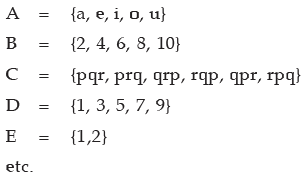This form is called Roster or Braces form. In this form we make a list of the elements of the set and put it within braces { }.
Instead of listing we could describe them as follows :

A = the set of vowels in the alphabet
B = The set of even numbers between 2 and 10 both inclusive.
C = The set of all possible arrangements of the letters p, q and r
D = The set of odd digits between 1 and 9 both inclusive.
E = The set of roots of the equation x2 – 3x + 2 = 0
Set B, D and E can also be described respectively as
B = {x : x = 2m and m being an integer lying in the interval 0 < m < 6}
D = {2x – 1 : 0 < x < 5 and x is an integer}
E = {x : x2 – 3x + 2 = 0}

This form is called set-Builder or Algebraic form or Rule Method. This method of writing the set is called Property method. The symbol : or/reads 'such that'. In this method, we list the property or properties satisfied by the elements of the set.

We write, {x:x satisfies properties P}. This means, "the set of all those x such that x satisfies the properties P".

A set may contain either a finite or an infinite number of members or elements. When the number of members is very large or infinite it is obviously impractical or impossible to list them all. In such case.

we may write as :
N = The set of natural numbers = {1, 2, 3…..}
W = The set of whole numbers = {0, 1, 2, 3,…)
etc.
I. The members of a set are usually called elements. In A = {a, e, i, o, u}, a is an element and we write a ∈A i.e. a belongs to A. But 3 is not an element of B = {2, 4, 6, 8, 10} and we write 3∉B. i.e. 3 does not belong to B.
II. If every element of a set P is also an element of set Q we say that P is a subset of Q. We write P ⊂ Q . Q is said to be a superset of P. For example {a, b} ⊂ {a, b, c}, {2, 4, 6, 8, 10} ⊂ N. If there exists even a single element in A, which is not in B then A is not a subset of B.
III. If P is a subset of Q but P is not equal to Q then P is called a proper subset of Q.
IV. ϕ has no proper subset.
Illustration: {3} is a proper subset of {2, 3, 5}. But {1, 2} is not a subset of {2, 3, 5}.

Thus if P = {1, 2} and Q = {1, 2 ,3} then P is a subset of Q but P is not equal to Q. So, P is a proper subset of Q.
To give completeness to the idea of a subset, we include the set itself and the empty set. The empty set is one which contains no element. The empty set is also known as null or void set usually denoted by { } or Greek letter ϕ , to be read as phi. For example the set of prime numbers between 32 and 36 is a null set. The subsets of {1, 2, 3,} include {1, 2, 3}, {1, 2}, {1, 3}, {2, 3}, {1}, {2}, {3} and { }.
A set containing n elements has 2n subsets. Thus a set containing 3 elements has 23 (=8) subsets.
A set containing n elements has 2n –1 proper subsets. Thus a set containing 3 elements has 23 –1 ( =7) subsets. The proper subsets of { 1,2,3} include {1, 2}, {1, 3}, {2, 3}, {1}, {2}, {3], { } .
Suppose we have two sets A and B. The intersection of these sets, written as A ∩ B contains

those elements which are in A and are also in B.
For example A = {2, 3, 6, 10, 15}, B = {3, 6, 15, 18, 21, 24} and C = { 2, 5, 7}, we have A ∩ B = { 3, 6, 15}, A ∩ C = {2}, B ∩ C = ф , where the intersection of B and C is empty set. So, we say B and C are disjoint sets since they have no common element. Otherwise sets are called overlapping or intersecting sets. The union of two sets, A and B, written as A ∪ B contain all these elements which are in either A or B or both.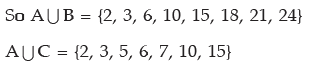A set which has at least one element is called non-empty set . Thus the set { 0 } is non-empty set.
It has one element say 0.
Singleton Set: A set containing one element is called Singleton Set.
For example {1} is a singleton set, whose only member is 1.
Equal Set: Two sets A & B are said to be equal, written as A = B if every element of A is in B

and every element of B is in A.
Illustration: If A = {2, 4, 6} and B = {2, 4, 6} then A = B.
Remarks : (I) The elements of a set may be listed in any order.
Thus, {1, 2, 3} = {2, 1, 3} = {3, 2, 1} etc.
(II) The repetition of elements in a set is meaningless.
Example: {x : x is a letter in the word "follow"} = {f, o, l, w}
Example: Show that ф , {0} and 0 are all different.
Solution: ф is a set containing no element at all; {0} is a set containing one element, namely 0.
And 0 is a number, not a set.
Hence ф ,{0} and 0 are all different.
The set which contains all the elements under consideration in a particular problem is called the universal set denoted by S. Suppose that P is a subset of S. Then the complement of P, written as Pc (or P') contains all the elements in S but not in P. This can also be written as S – P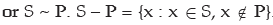For example let S = {0, 1, 2, 3, 4, 5, 6, 7, 8, 9}
P = {0, 2, 4, 6, 8}
Q = {1, 2, 3, 4, 5)
Then P' = {1, 3 ,5 ,7, 9} and Q'= {0 , 6 , 7, 8, 9}
Also P ∪ Q = {0, 1, 2, 3, 4, 5, 6, 8}, (P ∪ Q)1 = {7, 9}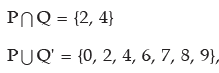(P ∩ Q)' = {0, 1, 3, 5, 6, 7, 8, 9}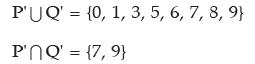So it can be noted that (P ∪ Q)' = P' ∩ Q' and (P ∩  Q)' = P' ∪Q'. These are known as De Morgan’s laws.
VENN DIAGRAMS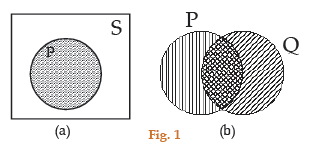Thus Fig. 1(a) shows a universal set S represented by a rectangular region and one of its subsets P represented by a circular shaded region.
The un-shaded region inside the rectangle represents P'.
Figure 1(b) shows two sets P and Q represented by two intersecting circular regions. The total shaded area represents PUQ, the cross-hatched "intersection" represents P ∩ Q.
The number of distinct elements contained in a finite set A is called its cardinal number. It is denoted by n(A). For example, the number of elements in the set R = {2, 3, 5, 7} is denoted by n(R). This number is called the cardinal number of the set R.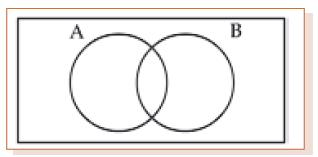Thus n(AUB) = n(A) + n(B) – n(A∩ B)

If A and B are disjoint sets, then n(AUB) = n(A) + n(B) as n (A∩ B) = 0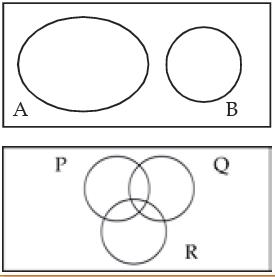For three sets P, Q and R
n(PUQUR) = n(P) + n(Q) + n(R) – n(P ∩ Q) – n(Q ∩ R) – n(P ∩ R) + n(P ∩ Q∩ R)
When P, Q and R are disjoint sets
= n(P) + n(Q) + n(R)
Illustration: If A = {2, 3, 5, 7} , then n(A) = 4
Equivalent Set: Two finite sets A & B are said to be equivalent if n (A) = n(B).
Clearly, equal sets are equivalent but equivalent sets need not be equal.
Illustration: The sets A = {1, 3, 5} and B = {2, 4, 6} are equivalent but not equal.
Here n (A) = 3 = n (B) so they are equivalent sets. But the elements of A are not in B. Hence they are not equal though they are equivalent.
Power Set: The collection of all possible subsets of a given set A is called the power set of A, to be denoted by P(A).

Illustration: (I) If A = {1, 2, 3} then
P(A) = { {1, 2, 3}, {1, 2}, {1, 3}, {2, 3}, {1}, {2}, {3}, ф }
(II) If A = {1, {2}}, we may write A = {1, B} when B = {2} then
P(A) = { ф , {1}, {B }, {1, B}} = { ф , {1}, {{2}}, {1,{2}}}

PRODUCT SETS
Ordered Pair: Two elements a and b, listed in a specific order, form an ordered pair, denoted by (a, b).
Cartesian Product of sets : If A and B are two non-empty sets, then the set of all ordered pairs (a, b) such that a belongs to A and b belongs to B , is called the Cartesian product of A and B, to be denoted by A × B.
Thus, A × B = {(a, b) : a ∈ A and b ∈ B}
If A = ф or B = ф , we define A × B = ф
Illustration: Let A = {1, 2, 3}, B = {4, 5}
Then A × B = { (1, 4), (1, 5), (2, 4) (2, 5), (3, 4), (3, 5) }
Example: If A × B = { ( 3, 2 ) , (3, 4) , (5, 2), (5, 4) } , find A and B.
Solution: Clearly A is the set of all first co-ordinates of A × B, while B is the set of all second co-ordinates of elements of A × B.
Therefore A = {3, 5} and B = {2 , 4}
Example: Let P = {1, 3, 6} and Q {3, 5}
The product set P × Q = {(1, 3), (1, 5), (3, 3), (3, 5), (6, 3), (6, 5)} .
Notice that n(P×Q) = n(P) × n(Q) and ordered pairs (3, 5) and (5, 3) are not equal.
and Q × P = {(3, 1), (3, 3), (3, 6), (5, 1), (5, 3), (5, 6)}
So P × Q ≠ Q × P; but n (P × Q) = n (Q × P).
Illustration: Here n(P) = 3 and n(Q ) = 2, n (P × Q) = 6. Hence n(P×Q) = n(P) × n(Q). and n(P × Q ) = n(Q × P) = 6.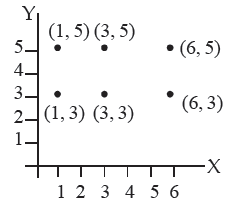We can represent the product set of ordered pairs by points in the XY plane

If X=Y= the set of all natural numbers, the product set XY represents an infinite equal lattice of points in the first quadrant of the XY plane.
RELATIONS AND FUNCTIONS
Any subset of the product set X.Y is said to define a relation from X to Y and any relation from X to Y in which no two different ordered pairs have the same first element is called a function.
Let A and B be two non-empty sets. Then, a rule or a correspondence f which associates to

each element x of A, a unique element, denoted by f(x) of B , is called a function or mapping from A to B and we write f : A→B
The element f(x) of B is called the image of x, while x is called the pre-image of f (x).

DOMAIN & RANGE OF A FUNCTION
Let f : A→B, then A is called the domain of f, while B is called the co-domain of f.
The set f(A) = {f (x) : x ∈ A} is called the range of f.
Illustration: Let A = {1, 2, 3, 4} and B ={1, 4, 9, 16, 25}
We consider the rule f(x) = x2 . Then f(1) = 1 ; f(2) =4 ; f (3) = 9 & f (4) = 16.
Then clearly each element in A has a unique image in B.
So, f : A→B : f (x) = x2 is a function from A to B.
Here domain of f = {1, 2, 3, 4} and range of f = {1, 4, 9, 16}
Example: Let N be the set of all natural numbers. Then, the rule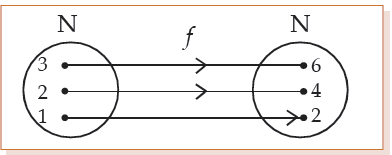f : N → N : f(x) = 2x, for all x ∈ N is a function from N to N, since twice a natural number is unique.
Now, f (1) = 2; f (2) = 4; f(3) = 6 and so on.
Here domain of f = N = {1, 2 ,3, 4 ,………..} ; range of f = { 2, 4, 6,………..}
This may be represented by the mapping diagram or arrow graph.
VARIOUS TYPES OF FUNCTION
One-One Function : Let f : A→B. If different elements in A have different images in B, then f is said to be a one-one or an injective function or mapping.
Illustration: (i) Let A = {1, 2, 3} and B = {2, 4, 6}
Let us consider f : A→B : f(x) = 2x.
Then f(1) = 2; f(2) =4 ; f (3) = 6
Clearly, f is a function from A to B such that different elements in A have different images in B.
Hence f is one-one.
Remark : Let f : A→B and let x1 , x2 ∈ A.
Then x1 = x2 implies f(x1) = f(x2) is always true.
But f(x1) = f(x2) implies x1 = x2 is true only when f is one-one.
(ii) let x={1, 2, 3, 4} and y={1, 2, 3}, then the subset {(1, 2), (1, 3), (2, 3)} defines a relation on X.Y.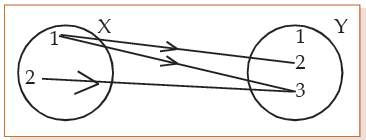Notice that this particular subset contains all the ordered pair in X.Y for which the X element (x) is less than the Y element (y). So in this subset we have X<Y and the relation between the set, is "less than". This relation is not a function as it includes two different ordered pairs (1, 2), (1, 3) have same first element.

X.Y={(1, 1), (1, 2), (1, 3), (2, 1), (2, 2), (2, 3)
(3, 1), (3, 2), (3, 3),(4, 1), (4, 2), (4, 3)}

The subset {(1, 1), (2, 2), (3, 3)} defines the function y= x as different ordered pairs of this subset have different first element.

 Onto or Surjective Functions : Let f : A→B. If every element in B has at least one pre-image in A, then f is said to be an onto function.If f is onto, then corresponding to each y ∈ B, we must be able to find at least one elementx ∈ A such that y = f (x)Clearly, f is onto if and only if range of f = B

Illustration: Let N be the set of all natural numbers and E be the set of all even natural numbers.
Then, the function
f : N → E : f (x) = 2x , for all x ∈ N
is onto, since each element of E is of the form 2x, where x ∈ N and the same is the

f-image of x ∈ N.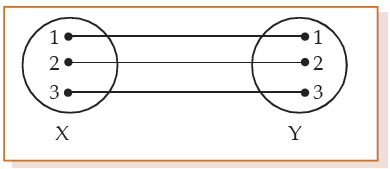Represented on a mapping diagram it is a one-one mapping of X onto Y.

 Bijection Function: A one-one and onto function is said to be bijective.A bijective function is also known as a one-to-one correspondence.Identity Function : Let A be a non-empty set. Then, the function I defined byI : A → A : I (x) = x for all x ∈ A is called an identity function on A.

It is a one-to-one onto function with domain A and range A.
Into Functions: Let f : A → B. There exists even a single element in B having no pre-image in A, then f is said to be an into function.
Illustration: Let A = {2, 3, 5, 7} and B = {0, 1, 3, 5, 7} . Let us consider f : A → B;
f (x) = x – 2. Then f(2) = 0; f(3) = 1 ; f (5) =3 & f(7) = 5.
It is clear that f is a function from A to B .
Here there exists an element 7 in B, having no pre-mage in A.
So, f is an into function.
Constant Function: Let f : A → B, defined in such a way that all the elements in A have the

same image in B, then f is said to be a constant function.

Illustration: Let A = {1, 2, 3} and B = {5, 7, 9}. Let f : A → B : f (x) = 5 for all x ∈ A.
Then, all the elements in A have the same image namely 5 in B.
So, f is a constant function.
Remark: The range set of a constant function is a singleton set.
Example: Another subset of X.Y is {(1, 3), (2, 3), (3, 3), (4, 3)}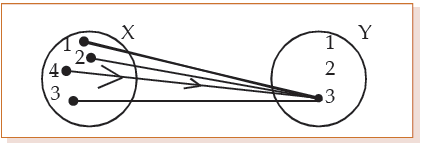This relation is a function (a constant function). It is represented on a mapping diagram and is a many-one mapping of X into Y.

Let us take another subset {(4, 1), (4, 2), (4, 3)} of X.Y. This is a relation but not a function. Here different ordered pairs have same first element so it is not a function.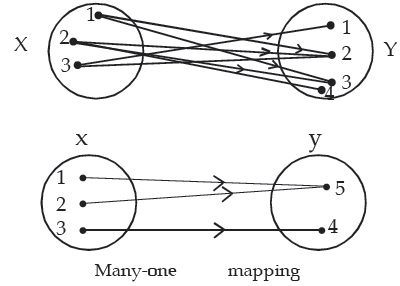This is an example of many-one mapping.

Equal Functions: Two functions f and g are said to be equal, written as f = g if they have the same domain and they satisfy the condition f(x) = g(x), for all x.

A function may simply pair people and the corresponding seat numbers in a theatre. It may simply associate weights of parcels with portal delivery charge or it may be the operation of squaring, adding to doubling, taking the log of etc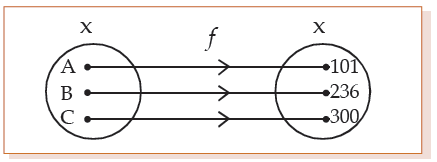The function f here assigning a locker number to each of the persons A, B and C. Names are associated with or mapped onto, locker numbers under the function f.
We can write f : X → Y or f(x) = y or f(B) = 236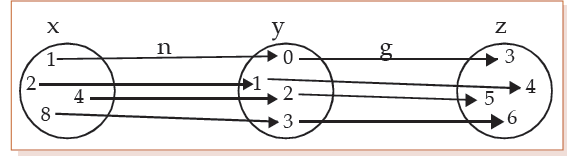This diagram shows the effect of two functions n and g on the sets X, Y and Z
n : X→Y and g : Y→Z
If x, y, z are corresponding elements of X, Y and Z, we write n(x) = y and g(y) = z
Thus n(1) = 0 and g (0) = 3, so that g (n(1)) = g(0) = 3 we can write it as gn(1) or gon (1) = 3 But g(1) = 4 and n(g(1) )= n(4) = 2
So gn ≠ ng (or, g o n ≠ n o g)
The function gn or ng is called a composite function. As n(8) = 3, we say that 3 is the image of 8 under the mapping (or function) n.

 Inverse Function: Let f be a one-one onto function from A to B. Let y be an arbitrary element of B. Then f being onto, there exists an element x in A such that f (x) = y.As f is one-one this x is unique.Thus for each y∈B, there exists a unique element x ∈ A such that f (x) = y.So, we may define a function, denoted by f –1 as:f–1 : B→A : f–1 (y ) = x if and only if f (x) = y.The above function f–1 is called the inverse of f.A function is invertible if and only if f is one-one onto.

Remarks: If f is one-one onto then f–1 is also one-one onto

Illustration: The relation "is parallel to" on the set S is
(1) reflexive, since a || a for a ∈ S
(2) symmetric, since a || b ⇒ b || a
(3) transitive, since a || b , b || c ⇒ a || c
Hence it is an equivalence relation.

 Domain & Range of a relation: If R is a relation from A to B, then the set of all first coordinates of elements of R is called the domain of R, while the set of all second co-ordinates of elements of R is called the range of R.So, Dom (R) = { a : (a, b) ∈ R } & Range ( R) = { b : (a, b) ∈ R}

Illustration: Let A = {1, 2, 3} and B = {2, 4, 6}
Then A × B = {(1, 2), (1, 4), (1, 6), (2, 2), (2, 4), (2, 6), (3, 2), (3, 4), (3, 6)}
By definition every subset of A × B is a relation from A to B.
Thus, if we consider the relation
R = { (1, 2), (1, 4), (3, 2), (3, 4)} then Dom (R) = {1, 3} and Range (R)= {2, 4}
From the product set X. Y = {(1, 3), (2, 3), (3, 3), (4, 3), (2, 2), (3, 2), (4, 2), (1, 1), (2, 1), (3, 1), (4, 1)}, the subset {(1, 1), (2, 2), (3, 3)} defines the relation 'Is equal to' , the subset {(1, 3), (2, 3), (1, 2)} defines 'Is less than', the subset {(4, 3), (3, 2), (4, 2), (2, 1), (3, 1), (4, 1)} defines 'Is greater than' and the subset {(4, 3), (3, 2), (4, 2), (2, 1), (3, 1), (4, 1), (1, 1), (2, 2) (3, 3)} defines to greater 'In greater than or equal'.

Illustration: Let A = {1, 2, 3} and b = {2, 4, 6}

Then A × B = {(1, 2), (1, 4), (1, 6), (2, 2), (2, 4), (2, 6), (3, 2), (3, 4), (3, 6)}

If we consider the relation = {(1, 2), (1, 4), (3, 4)} then Dom (R) = {1, 3} and

Range = {2, 4}. Here the relation "Is less than".

Identity Relation: The relation I = {(a, a) : a ∈A} is called the identity relation on A.

Illustration:Let A = {1, 2, 3} then I = {(1, 1), (2, 2), (3, 3)}

Inverse Relation: If R be a relation on A, then the relation R–1 on A, defined by

R–1 = {(b, a) : (a, b) ∈ R} is called an inverse relation on A.

Clearly , Dom (R–1) = Range (R) & Range (R–1) = Dom (R).

Illustration: Let A = {1, 2, 3} and R = {(1, 2), (2, 2), (3, 1), (3, 2)}
Then R being a subset of a × a, it is a relation on A. Dom (R) = {1, 2, 3} and Range (R) = {2, 1}
Now, R–1 = {(2, 1), (2, 2), (1, 3), (2, 3)}. Here, Dom (R–1) = {2, 1} = Range (R) and

Range (R–1 ) = {1, 2, 3} = Dom (R).
Illustration: Let A = {1, 2, 3}, then
(i) R1 = {(1, 1), (2, 2), (3, 3), (1, 2)}

Is reflexive and transitive but not symmetric, since (1, 2) ∈ R1 but (2, 1) does not belongs to R1.
(ii) R2 = {(1, 1), (2, 2), (1, 2), (2, 1)}
is symmetric and transitive but not reflexive, since (3, 3) does not belong to R2.
(iii) R3 = {(1, 1), (2, 2), (3, 3), (1, 2), (2, 1), (2, 3), (3, 2)} is reflexive and symmetric but not transitive , since (1, 2) ∈ R3 & (2, 3) ∈ R3 but (1, 3) does not belong to R3.
Problems and solution using Venn Diagram
1. Out of a group of 20 teachers in a school, 10 teach Mathematics, 9 teach Physics and 7

teach Chemistry. 4 teach Mathematics and Physics but none teach both Mathematics and Chemistry. How many teach Chemistry and Physics? How many teach only Physics ?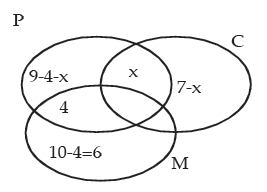Let x be the no. of teachers who teach both Physics & Chemistry.
9 – 4 – x + 6 + 7 – x + 4 + x = 20
or 22 – x = 20
or x = 2
Hence, 2 teachers teach both Physics and Chemistry and 9 – 4 – 2 = 3 teachers teach only

Physics.
2. A survey shows that 74% of the Indians like grapes, whereas 68% like bananas.

What percentage of the Indians like both grapes and bananas?
Solution: Let P & Q denote the sets of Indians who like grapes and bananas respectively.

Then n(P ) = 74, n(Q) = 68 and n(P∪ Q) = 100.

We know that n( P∩ Q) = n(P) + n(Q) – n( P ∪ Q ) = 74 + 68 – 100 = 42.

Hence, 42% of the Indians like both grapes and bananas.

3. In a class of 60 students, 40 students like Maths, 36 like Science, and 24 like both the

subjects. Find the number of students who like

(i) Maths only
(ii) Science only
(iii) either Maths or Science
(iv) neither Maths nor Science
Solution: Let M = students who like Maths and S = students who like Science
Then n( M) = 40, n(S) = 36 and n (M∩ S ) = 24
Hence,
(i) n(M) – n(M∩ S) = 40 – 24 = 16 = number of students like Maths only.
(ii) n( S ) – n(M∩ S) = 36 – 24 = 12 = number of students like Science only.
(iii) n(M∪ S) = n(M) + n(S) – n(M ∩ S) = 40 + 36 – 24 = 52 = number of students who like either Maths or Science.
(iv) n(M∪ S)c = 60 – n(M∪ S ) = 60 – 52 = 8 = number of students who like neither Maths nor Science.

Offer running on EduRev: Apply code STAYHOME200 to get INR 200 off on our premium plan EduRev Infinity!

## Business Mathematics and Logical Reasoning & Statistics

33 videos|101 docs|87 tests

,

,

,

,

,

,

,

,

,

,

,

,

,

,

,

,

,

,

,

,

,

,

,

,

;